# Merge a linked list into another linked list at alternate positions

## Introduction to the problem statement

Let us look at what the problem says:

Given two singly-linked lists, merge the second list into the first list at alternating positions; if the second link list includes extra nodes, print them as well. The nodes in the second list will be inserted only when positions are available.

Example 1:-

Explanation:-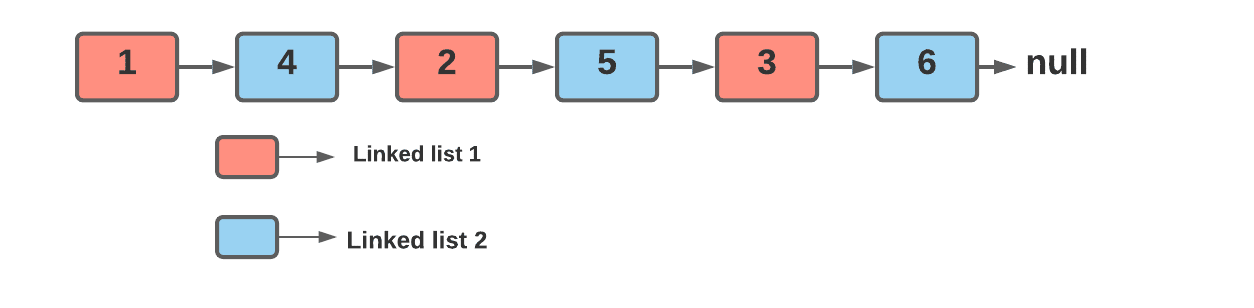Example 2:-

Explanation:-

The nodes of the second list should only be inserted when there are positions available in the first list and here the second list has more nodes than the first. To put it simply, the second list can only be inserted if the nodes in it are less than or equal to the nodes in the first list.

As a result, the output is as follows.Example 3:-

Explanation:-

Since the first list is empty the output will be printed for the second list.Example 4:-

Explanation:-

In this case, the second list is empty, thus, the output is printed for the first list.Example 5:-

Explanation:-Before we move further to the approach let us recall some properties of linked list data structure:

What is a linked list data structure?

• A LinkedList is a collection of elements called nodes that are stored in memory at random.
• A node has two fields: data saved at that specific address and a pointer containing the address of the next node in memory.
• The list's last node contains a pointer to the null.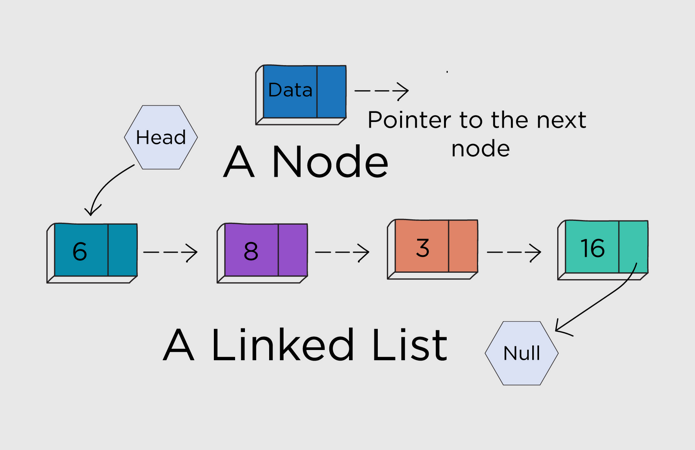Source: Vivosoft

In programming, there are many different types of linked lists, but we generally refer to the singly linked list, which is what we've examined in the preceding paragraphs.

Let us now get back to the problem:-

The idea is very simple, if we look closely at the problem examples then it is clear that we need to update the links of the nodes by taking care of the corresponding nodes.

We'll simply run a loop while there are open positions in the first linked list and insert nodes from the second list between the first by altering pointers.

## Approach

• Assume Nodes A and B are the beginnings (heads) of two linked lists.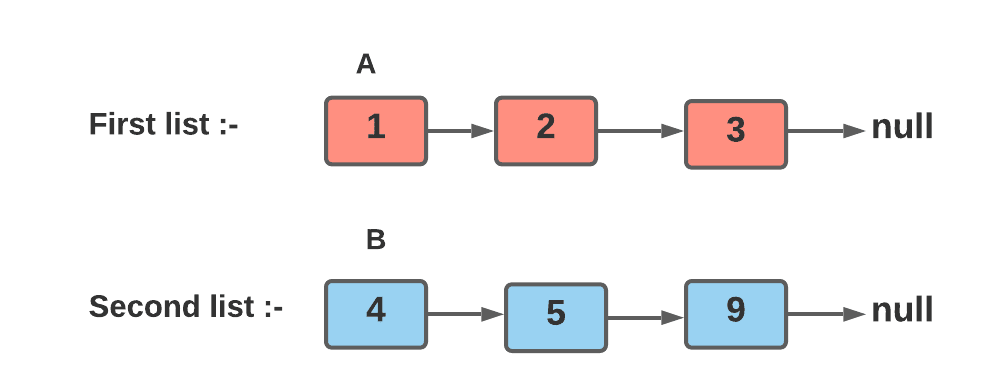• Take Node result = A ( store the head of the link list one).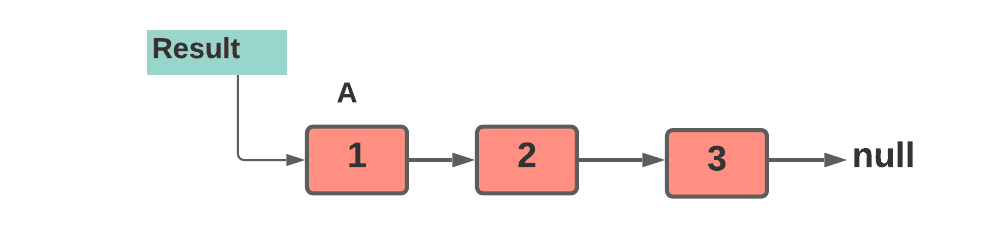• Make Node node1next= A.next and Node node2next = B.next.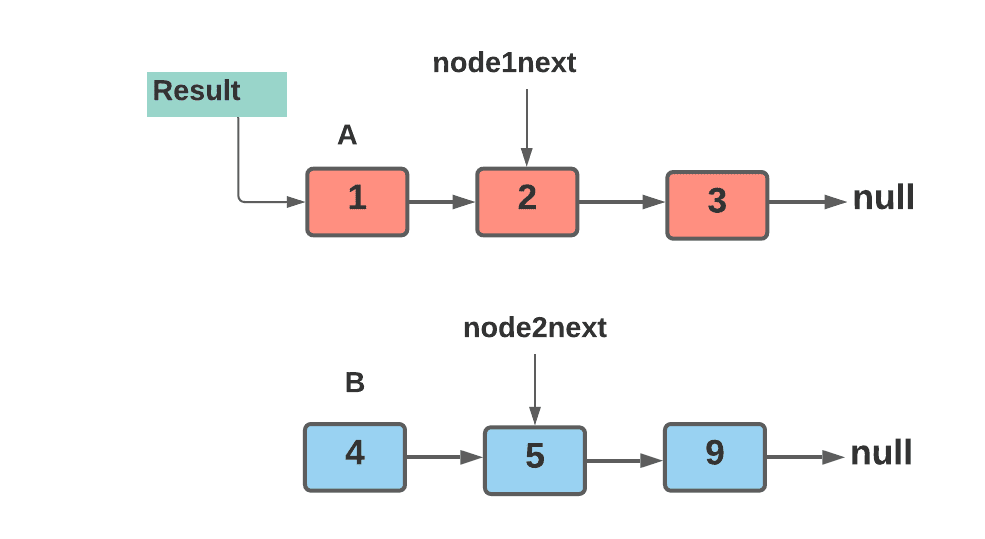• Update the link of node 1.

A.next = B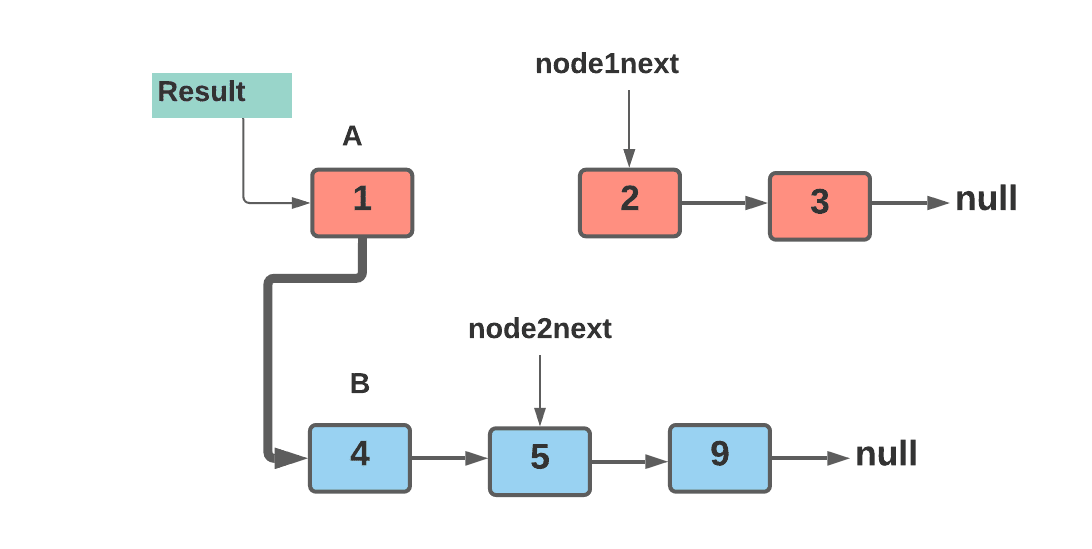• Make B.next = node1next. (At this point, B is situated between A and node1next).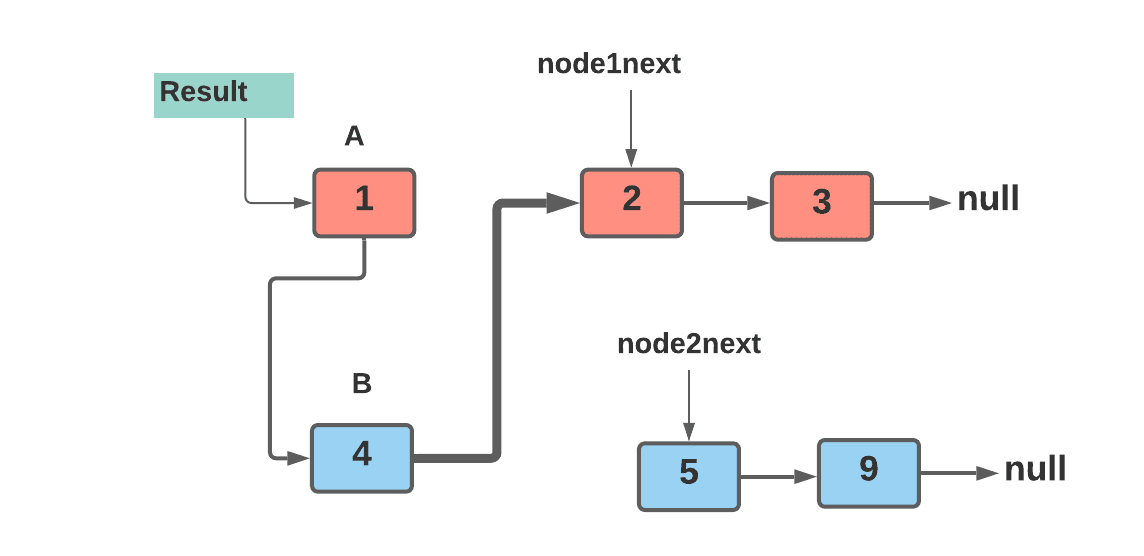• The A pointer should be updated to point to the next node in the first linked list.

A = node1next.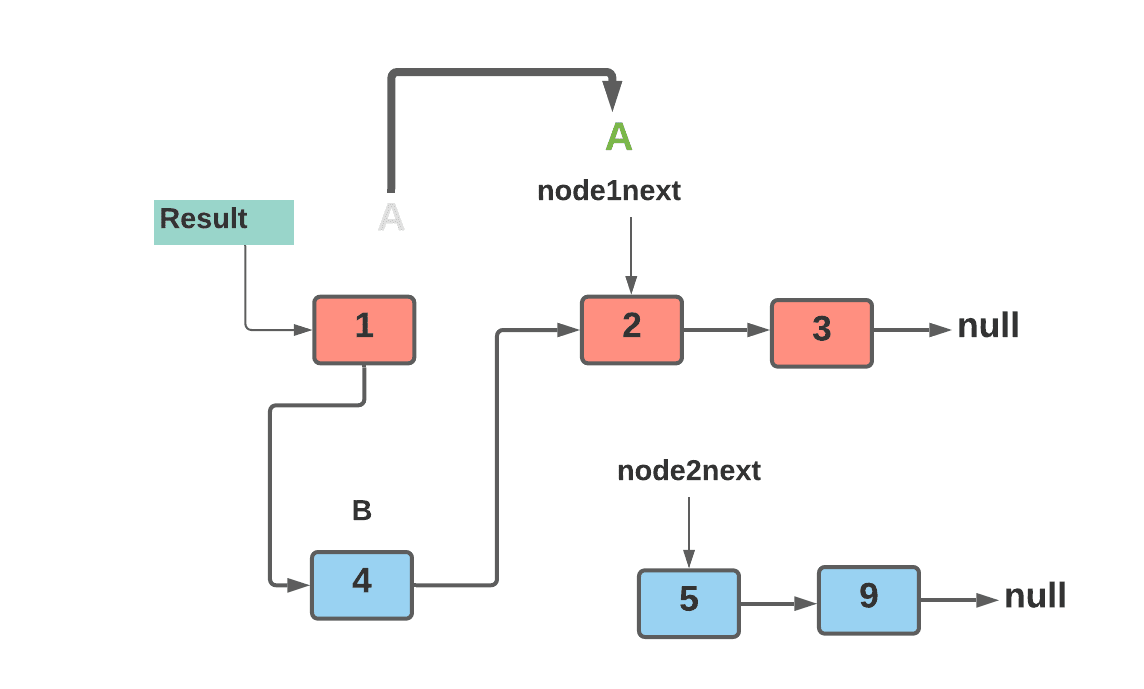• The B pointer should be updated to point to the next node in the second linked list.

B = node2next.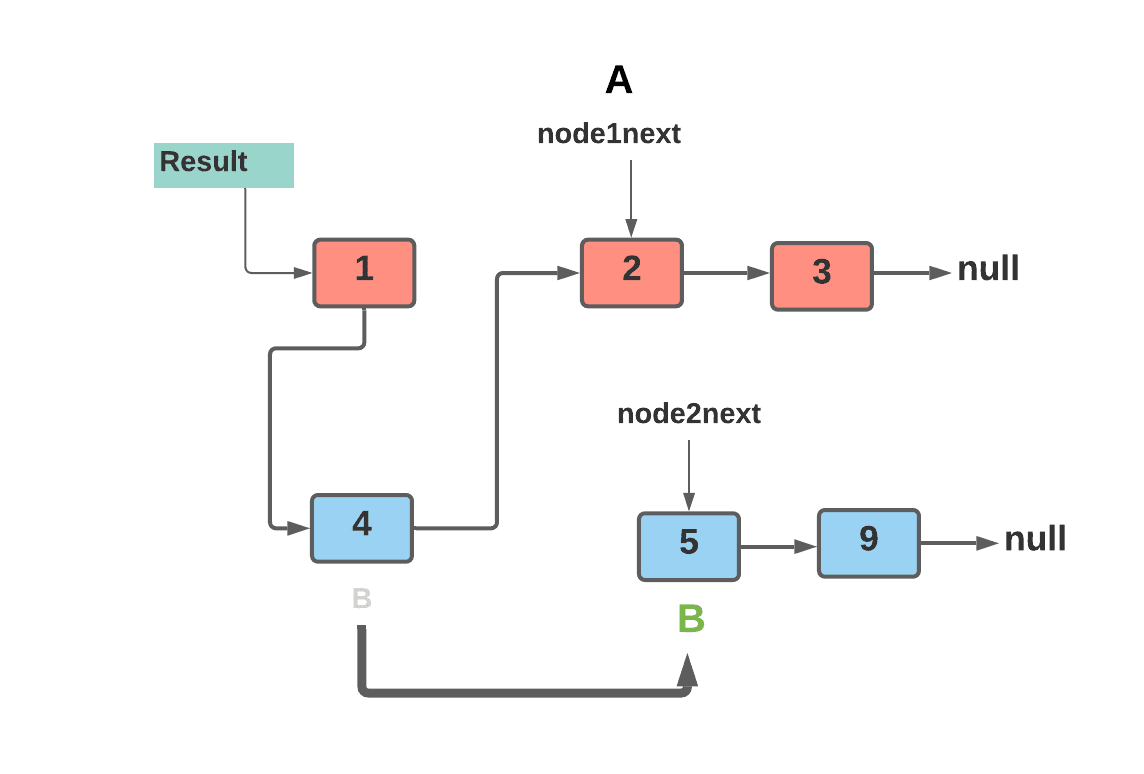• Repeat the previous two procedures until one of the items on the list burns out.
• Using the result node, print the list.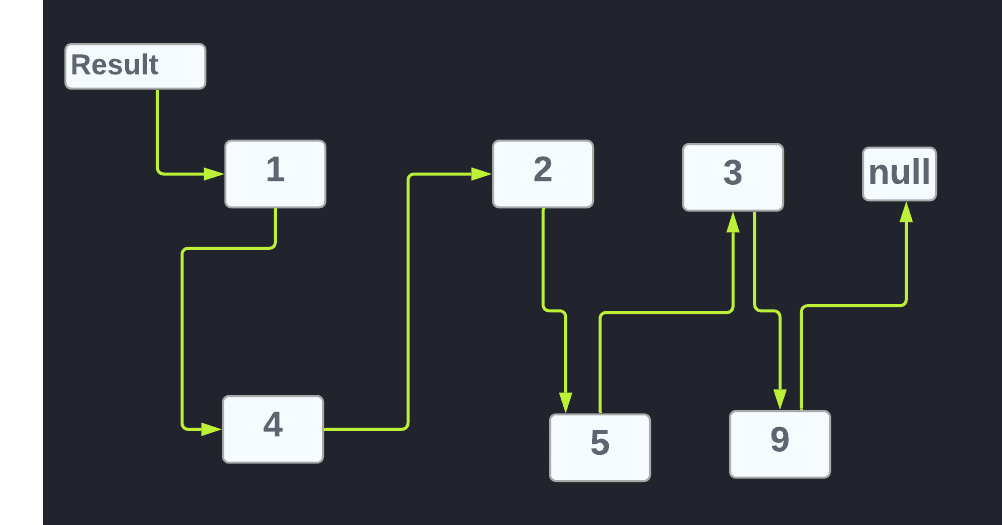• Print the remaining list in B, if any, with B pointing to the top of the list.

## Pseudo Code

``````public void mergeList(Node A, Node B)
Node result = A
while (A != null and B != null)
Node node1next = A.next
Node node2next = B.next
A.next = B
B.next = node1next
A = node1next
B = node2next
}
printList(result)``````

## Implementation in Java

``````// Java program -> to merge a linked list into another linked list at alternative positions
import java.util.*;
import java.io.*;

// Node class to create the new node, having two fields:- 1. data 2. next to point the next node
class Node {  public int data;
public Node next;
// function to assign the provided data value to the new node
public Node(int data) {
this.data = data;
this.next = null;
}

}

public class MergeTwoListAtAlternatePositions {

// Utility function to print the linked list
while (currNode != null) {
System.out.print(currNode.data + "->");
currNode = currNode.next;
}
System.out.print("null");
}

// function to merge a linked list into another
public void mergeList(Node A, Node B) {

// result node for pointing the head of the new list
Node result = A;

// while loop to traverse through the linked list until the cursor is pointing to the null
while (A != null && B != null) {

// node1next to keep the track on immediate node of the current head node of first linked list
// for example:- if we have L1= 1->2->3->null , L2= 4->5->->null
// In order to update the links, i.e, Node 1 has to pair with Node 4,( 1->4). then Node 4 has to pair with
// Node 2 in the first linked list, we woud be needing an additional node who will point to the every next node
Node node1next = A.next;

// node2next to keep the track on immediate node of the current head node of second linked list
Node node2next = B.next;

// Join the second list's node with first linked list
// eg:- L1= 1->2->3->null L2 = 4->5->6->null
// It has to be 1->4..... then so on
A.next = B;

// Since now, 1->4, now it has to join with the next node
// i.e, 1->4->2->...... so on.
B.next = node1next;

A = node1next;
B = node2next;
}
System.out.println("\n Merge a linked list into another:- ");
// print the updated list
printList(result);
System.out.println("\nRemaining Second List if any");
// B will point to the head of the remaining list
printList(B);

}
// main function
public static void main(String[] args) throws java.lang.Exception {
// create the object of public class MergeTwoListAtAlternatePositions
MergeTwoListAtAlternatePositions obj = new MergeTwoListAtAlternatePositions();

// 1->2->3->4->5
Node A = new Node(1);
A.next = new Node(2);
A.next.next = new Node(3);
A.next.next.next = new Node(4);
A.next.next.next.next = new Node(5);
// print the first linked list
obj.printList(A);
System.out.println("");

// 6->7->8->9->10->11
Node B = new Node(6);
B.next = new Node(7);
B.next.next = new Node(8);
B.next.next.next = new Node(9);
B.next.next.next.next = new Node(10);
B.next.next.next.next.next = new Node(11);
// print the second linked list
obj.printList(B);
// finally merge the second one into one
obj.mergeList(A, B);
}
}``````

Output

``````1->2->3->4->5->null
6->7->8->9->10->11->null
Merge a linked list into another:-
1->6->2->7->3->8->4->9->5->10->null
Remaining Second List if any
11->null``````

### Complexity Analysis

Time Complexity:  The time complexity for the above approach is O(m+n), where m and n are the total number of nodes in the first and second linked list.

Space Complexity: No additional space is used to implement the above approach.

1. What is the difference between a singly linked list and a doubly-linked list?
The traversal of elements in a singly linked list is limited to one direction. In contrast, a doubly-linked list allows for element traversal in both directions.

2. In which area of the memory, a linked list is saved?
Linked lists are created using dynamic memory allocation, say malloc. The heap is used for dynamic memory allocations. The heap is a global resource that contains all of the system's free memory. The heap is treated as a linked list of unused memory blocks, known as the free-list.

3. How much memory does a linked list use?
LinkedList only utilises the nodes it needs, which can be as small as 24 bytes.

4. What are the real-time applications of linked-lists?
Image viewer - Since the previous and next images are linked, they can be accessed using the next and previous buttons.
Previous and next page in a web browser - Since they are linked as a linked list, we may access the previous and next URL searched in a web browser by hitting the back and next buttons.
Music Player - The previous and next songs in the music player are linked. You can play songs from the beginning or finish of the list.

## Key takeaways

To summarise the talk, we looked at the problem approach, Merge a linked list into another linked list at alternating positions. Since the problem was related to a linked list, don't stop here; address other problems relating to the linked list:-

Similar problems you can look upon:-

Furthermore, we have a variety of well-structured courses just for you. So, what are you waiting for, get yourself enrolled now.